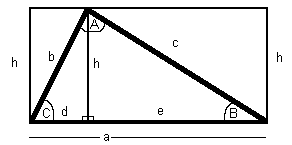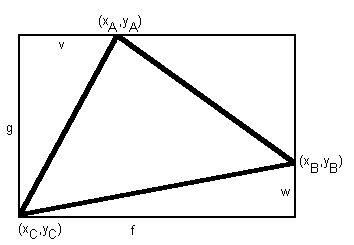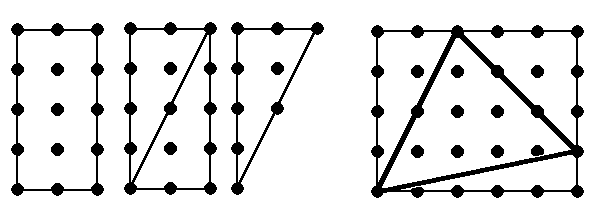# Area of a triangle

## Here are seven (slightly) different ways to calculate the area of a triangle:

 1. Area = a*h/22. Area = a*b*sin(C)/2 3. Area = a2*sin(B)*sin(C)/(2*sin(B+C)) 4. Area = sqrt(s*(s-a)*(s-b)*(s-c)) where s=(a+b+c)/2 is the semi-perimeter.5. Area = f*g/2 -v*w/26. Area = abs((xB*yA-xA*yB)+(xC*yB-xB*yC)+(xA*yC-xC*yA))/2If the vertices are at integer points on a grid of points then there is another: 7. Area = number of points inside triangle + half number of points on edge of triangle -1

## Proofs

### 1. Area = a*h/2

h is the height of the altitude of the triangle with base a.

The big rectangle is made up of two smaller rectangles with areas d*h and e*h. So the big triangle is made up of two smaller right-angled triangles whose areas are half of the smaller rectangles, i.e. d*h/2 and e*h/2. But a=d+e so the area of the large triangle is d*h/2+e*h/2 = a*h/2.

If one of the angles on the base is obtuse then the proof involves subtracting one half-rectangle from another.### 2. Area = a*b*sin(C)/2

h=b*sin(C) so using the result of 1 above gives the area of the triangle as a*b*sin(C)/2.### 3. Area = a2*sin(B)*sin(C)/(2*sin(B+C))

In the result of 2, there was nothing special about which angle and adjacent lines were used, since the same area will result, so we have Area = a*b*sin(C)/2 = b*c*sin(A)/2 = c*a*sin(B)/2.

This in turn leads to the sine rule: a*b*c/(2*Area) = a/sin(A) = b/sin(B) = c/sin(C). [This turns out to be the diameter of the circumcircle of the triangle (not proved here) so if this was D then the Area would be a*b*c/(2*D) = D2*sin(A)*sin(B)*sin(C)/2, providing two extra formulae.]

So we can use b=a*sin(B)/sin(A) to get the area of the triangle as a2*sin(B)*sin(C)/(2*sin(A)).

However A=p-B-C and sin(p-X)=sin(X) so sin(A)=sin(B+C),
giving the result that the area of the triangle is a2*sin(B)*sin(C)/(2*sin(B+C)).### 4. Area = sqrt(s*(s-a)*(s-b)*(s-c)) where s=(a+b+c)/2 is the semi-perimeter.

From the diagram (and Pythagoras's theorem) we have a=d+e, b2=h2+d2, and c2=e2+h2.
So d=sqrt(b2-h2),
and c2 =(a-d)2+h2=a2-2*a*d+b2=a2-2*a*sqrt(b2-h2)+b2,
which gives h=sqrt(4*a2*b2-(a2+b2-c2)2)/(2*a)
and with some rearrangement h=sqrt((a+b+c)*(-a+b+c)*(a-b+c)*(a+b-c))/(2*a).

But from the result of 1, the area of the triangle is a*h/2,
i.e. sqrt((a+b+c)*(-a+b+c)*(a-b+c)*(a+b-c))/4,
i.e. sqrt(((a+b+c)/2)*((-a+b+c)/2)*((a-b+c)/2)*((a+b-c)/2)),
which is sqrt(s*(s-a)*(s-b)*(s-c)) when s=(a+b+c)/2.

This result is called Heron's formula.

It could be rewritten as sqrt(2*(a2*b2+b2*c2+c2*a2)-(a4+b4+c4))/4.### 5. Area = f*g/2 -v*w/2

The area of the main triangle is the area of the enclosing rectangle less the three external right angled triangles,
i.e. f*g-f*w/2-v*g/2-(f-v)*(g-w)/2, [thanks to Gustavo Lau for correcting an error here]
which is f*g/2-v*w/2.

This gets more complicated if the three vertices are not all on the enclosing rectangle (because one of the angles is obtuse); but the result holds even in this case where the sides of the enclosing rectangle become f+v and g+w and one of the sides of the triangle is a diagonal of the rectangle.### 6. Area = abs((xB*yA-xA*yB)+(xC*yB-xB*yC)+(xA*yC-xC*yA))/2

Following 5, we have f=xB-xC, g=yA-yC, v=xA-xC and w=yB-yC.

So from the result of 5 the area of the triangle is (xB-xC)*(yA-yC)/2-(xA-xC)*(yB-yC)/2.
But this can be negative, depending on the direction in which the angles are taken,
so taking the absolute value and rearranging
we can get the area as abs((xB*yA-xA*yB)+(xC*yB-xB*yC)+(xA*yC-xC*yA))/2.

By adding triangles together, the result also extends to any simple polygon.### 7. If the vertices are at integer points on a grid of points then: Area = number of points inside triangle + half number of points on edge -1

For a rectangle whose edges of length x and y follow the grid, the area is x*y. The number of points strictly inside the rectangle is (x-1)*(y-1), while the number of points on the edge is 2*x+2*y.
Since (x-1)*(y-1)+(2*x+2*y)-1=x*y the result is true for rectangles of this form.

For a right angled triangle whose shorter edges of length x and y follow the grid, the area is x*y/2, since it and its congruent rotation together make up the earlier rectangle split by a diagonal. The number of points strictly inside the right angled triangle is (x-1)*(y-1)/2-z/2 where z is the number on the diagonal (but not in the vertices), while the number on the edge is x+y+z+1.
Since (x-1)*(y-1)/2-z/2+(x+y+z+1)-1=x*y/2 the result is true for right angled triangles of this form.

We can use this splitting tecnique to subtract any number of such right angled triangles from such a rectangle, without them having to be half the rectangle, since the area of the remaining shape plus that of the right angled triangles is that of the original recatangle. The number (counted with multiplicity) of points on the edge of the remaining shape and points on the edges of the right angled triangles is equal to the sum of the number of points on the edge of the rectangle, of twice the points (counted without multiplicity) newly on an edge, and of twice the number of right angled triangles (to reflect the effect of multiple counting of the verices on the diagonals of the right angled triangles). The number of points inside the the remaining shape and inside the right angled triangles is equal to the number of points inside the rectangle less the number (counted without multiplicity) newly on an edge. So for the remaining shape, which is this case is a general triangle, the result holds that the area is the number of points inside triangle plus half of the number of points on the edges minus one.

By adding triangles together, the result also extends to any simple polygon with its vertices at integer points on a grid of points.